# Determine if a sheet exists in a workbook using VBA in Microsoft Excel

There could be a time when you have to check if a worksheet, which you have create or deleted in a workbook in a VBA macro / code, exists. We can do this easily using a function / macro. There are multiple ways of checking if a worksheet exists.

1. User Defined Function known as UDF
2. Sub routine through message box

First option: User Defined Function

Following snapshot contains few sheets names & we will check if the names of sheet in column A exist.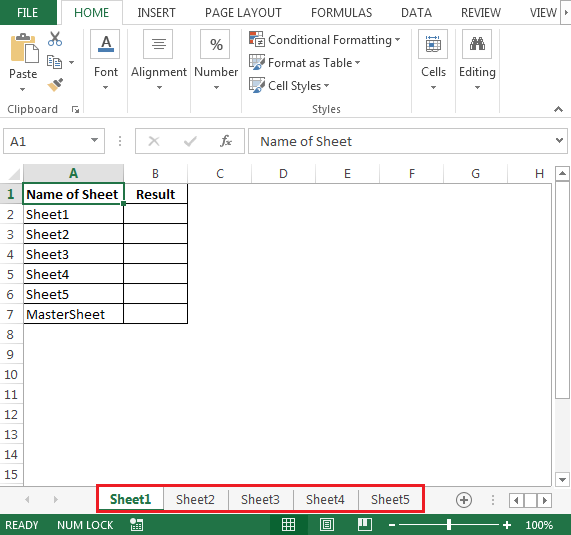To find if a specific sheet exists, we need to follow the below steps to launch VB editor

• Click on Developer tab
• From Code group select Visual Basic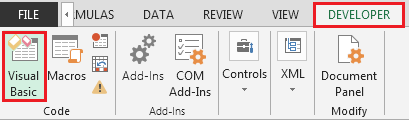• Copy the below code in the standard module

```Option Explicit

Function WorksheetExists(ByVal WorksheetName As String) As Boolean
Dim Sht As Worksheet

For Each Sht In ThisWorkbook.Worksheets
If Application.Proper(Sht.Name) = Application.Proper(WorksheetName) Then
WorksheetExists = True
Exit Function
End If
Next Sht
WorksheetExists = False
End Function
```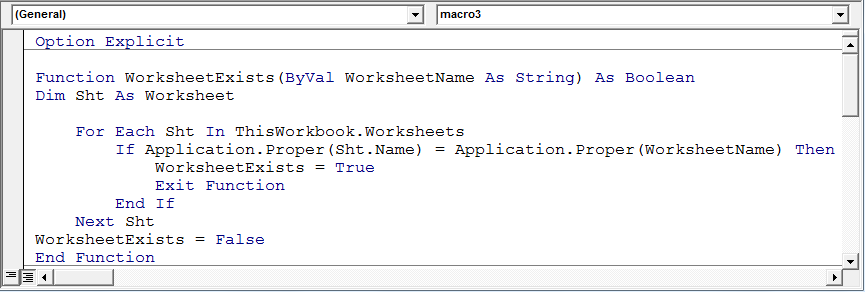• In order to check, we will use UDF in cell B2 as
• =WorksheetExists(A2)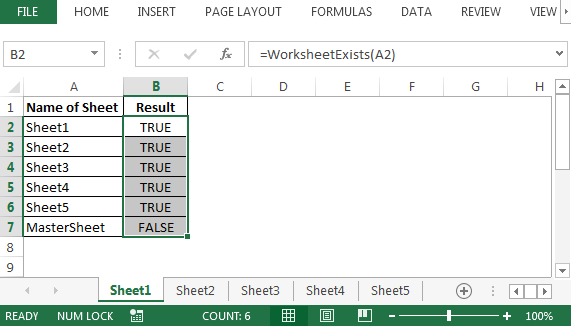• In the above image, “MasterSheet” does not exist in our sample workbook; hence, formula has given answer as False

Code Explanation:

This function takes the value for “WorksheetName” from the macro which performs other activities. If you need to change it as per your code, you may.

For Each Sht In ThisWorkbook.Worksheets and Next Sht are the starting and ending parts of the loop respectively.

Then If Application.Proper(Sht.Name) = Application.Proper(WorksheetName) Then

WorksheetExists = True

Checks if the Sheet name is matching the Sheet name passed from the main macro. If it does, WorksheetExists is True, and we can exit the function. Otherwise, WorksheetExists = False is returned back to the main macro. The loop goes from the 1st sheet to the next until all the sheets have been checked.

Second Option: Sub routine through message box

We can have a normal subroutine which is calling a UDF and, if the specified sheet is found, the message box will display, ‘sheet exist’; if not found, then msgbox pops up, ‘sheet not found’.

To check, we will copy the following code in the standard module:

```Function WorksheetExists2(WorksheetName As String, Optional wb As Workbook) As Boolean
If wb Is Nothing Then Set wb = ThisWorkbook
With wb
On Error Resume Next
WorksheetExists2 = (.Sheets(WorksheetName).Name = WorksheetName)
On Error GoTo 0
End With
End Function

Sub FindSheet()
If WorksheetExists2("Sheet1") Then
MsgBox "Sheet1 is in this workbook"
Else
MsgBox "Oops: Sheet does not exist"
End If
End Sub
```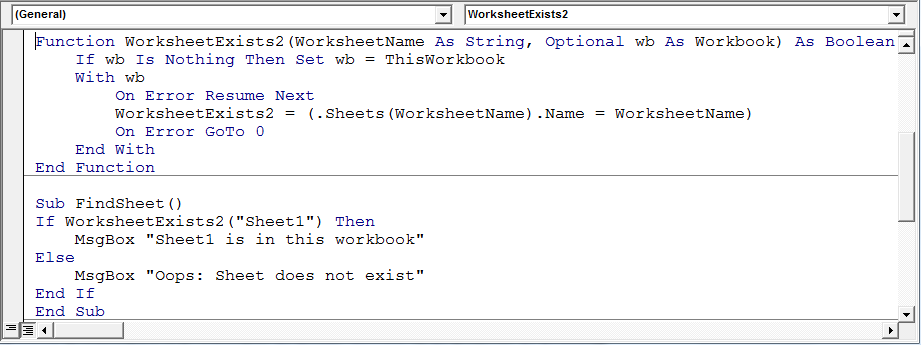After running the macro “FindSheet”, we will get the following message box if sheet exists: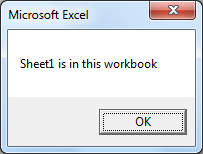If Sheet does not exist we will get the following message box: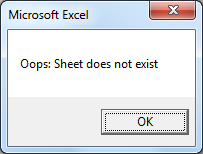Similarly, we can have a simple IF loop which checks if the sheet exists and performs certain actions thereafter.

```Sub test()
Dim ws As Worksheet
For Each ws In ThisWorkbook.Worksheets
If ws.Name <> "Main" Then
ws.Range("A1").Value = ws.Name
Else
End If
Next ws
End Sub
```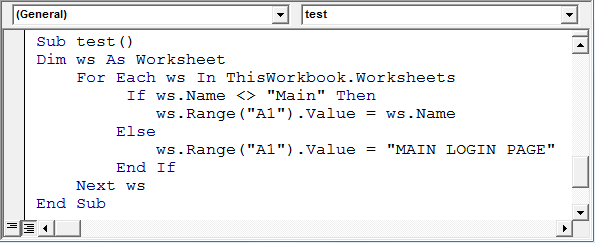• To test the above macro, we need to create a sheet name “Main”. This macro is very simple
• It loops through each worksheet in the workbook
• Then it checks if the worksheet name is not MAIN
• If it is MAIN, it displays text, like “MAIN LOGIN PAGE” in A1 of that sheet, else it displays the name of the sheet in cell A1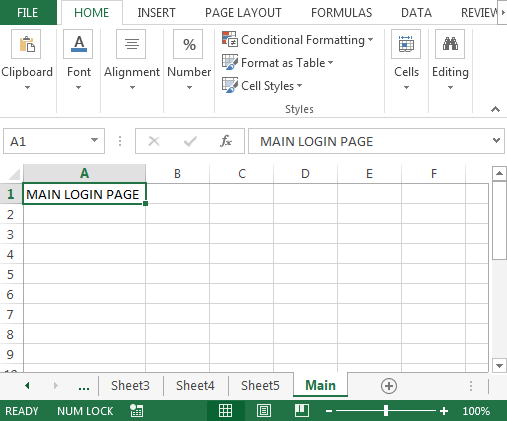• This is just another way of checking if the sheet exists. If it exists, perform action A, if not, action B

Conclusion: We can identify whether a sheet exists in our workbook or not; we can use UDF or subroutine as per our convenience.We would love to hear from you, do let us know how we can improve, complement or innovate our work and make it better for you. Write us at info@exceltip.com

1.Leigh Robertson

I need some code in the macro below to exit the sub if the sheet has already been created.
I must be getting too old I can't get my mind around it ......thanks Robbo

Sub CopyBingosheet()
'
' CopyBingosheet Macro
' Copy blank sheet and rename as the date
'

'

Sheets("Summary Sheet").Visible = True
Sheets("bingo sheet").Visible = True
Sheets("Summary Sheet").Select
Sheets("bingo sheet").Select
Sheets("bingo Sheet").Copy After:=ActiveWorkbook.Sheets(ActiveWorkbook.Sheets.Count)
Sheets("bingo sheet (2)").Select
Sheets("Bingo Sheet (2)").Name = Format(Date, "dd-mm-yyyy")

Range("Q16").Select
ActiveCell.FormulaR1C1 = "=TODAY()"
Sheets("bingo sheet").Select
ActiveWindow.SelectedSheets.Visible = False
Range("A6").Select
ActiveWorkbook.Save
End Sub

2.I want to Copy the data related to multiple Accounts into the different workbook which contains the Multiple tabs Account wise into the respective account tab,

3.Is there a reason this needs to be done this way? I use this within a Sub AddSheet() and it works @here:

Answer = Application.InputBox("Enter new Sheet name")
If Answer = "False" Then Exit Sub
On Error GoTo Continue:
MsgBox "Sheet Exists"
Exit Sub
Continue:

4.Hi,

I need support for, i have many excel workbook in a folder need to open on by one to take some data in one particular sheet which contain many 3 or 4 sheet, for ex.... data fetching from "My name" sheet if this sheet does not exist how to close this workbook and go to open next one same way continuously.... pls tell me code for this.

5.Although your solution works, you should never use errors to control the flow of a program. A more correct approach is:

Function WorksheetExists(ByVal WorksheetName As String) As Boolean
Dim Sht As Worksheet

For Each Sht In ThisWorkbook.Worksheets
If Application.Proper(Sht.Name) = Application.Proper(WorksheetName) Then
WorksheetExists = True
Exit Function
End If
Next Sht

WorksheetExists = False
End Function

Terms and Conditions of use

The applications/code on this site are distributed as is and without warranties or liability. In no event shall the owner of the copyrights, or the authors of the applications/code be liable for any loss of profit, any problems or any damage resulting from the use or evaluation of the applications/code.